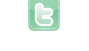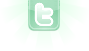## Variables/arrays help needed

Hello, I'm still working on my tic tac toe game. The placing in of crosses and naughts only if possible is working, but now I'm getting stuck on how to do the detection when someone won. I am looking at 2 dimensional arrays to store the coords of the placed crosses and circles, but I don't really know how to use them, and how to loop through them to check if f.e. 3 x-coordinates are the same for a cross, which would indicate the player with the crosses won. I also have an other questions, unrelated to above: How can you make like a pop up message that says "Cross won", and a button to restart? Thanks in advance for looking into this!
With x and y in range 0 thru 2:
```public boolean doesWin(int x, int y)
{
if (arr[y][x] == arr[y][(x+1)%3] && arr[y][x] == arr[y][(x+2)%3]) return true;
if (arr[y][x] == arr[(y+1]%3][x] && arr[y][x] == arr[(y+2)%3][x]) return true;
if (x+y == 2 & arr[y][x] == arr[(y+1)%3][(x+2)%3] && arr[y][x] == arr[(y+2)%3][(x+2)%3]) return true;
if (x == y && arr[y][x] == arr[(y+1)%3][(x+1)%3] && arr[y][x] == arr[(y+2)%3][(x+2)%3]) return true;
return false;
}```
If array holds Actor objects of different types, test class equivalences. If array hold one Actor type that has a field to indicate naught or cross, you will have to compare the field values. Note: it would be real easy to use 0, 1 and -1 (or 2) for "empty", "naught" and "cross" (or vice-versa). Then the above code would be exactly correct, if I did it right (and no Actor objects would be required; just update background of world). As far as winning status and button, the text image can either be directly drawn onto the background of the world or an image of an Actor object placed into the world; the button image should be an Actor object image. The world can use its presence to prevent game play and test for clicks on the actor.
Thank you for replying. I copied the code, and changed the +1 or +2 to +200 and +400 as my world is 600x600, and the cross or circle moves with 200 every time. I am getting a NullPointerException though. I declared the variable "private int arr;" in the beginning, and in the act method I have
`if(doesWin(e,f)) setImage("square.png")`
with e and f being the position of the cross.
Use:
`doesWin(e/200, f/200)`
for the condition (with +1 and +2 in the method).
I am still getting the NullPointerException. I read on Internet that that means that the array is not properly declared or something like that, but I don't see how to solve this immediatly? Thank you very much for helping!
`        int[][] arr = { {y}, {x}};`
in the method doesWin(int x, int y) and now I don't get the nullpointerexception anymore, but I get this error: java.lang.ArrayIndexOutOfBoundsException: Index 1 out of bounds for length 1 at Selector.doesWin(Selector.java:166) at Selector.act(Selector.java:76)
Windeycastle wrote...
`        int[][] arr = { {y}, {x}};`
in the method doesWin(int x, int y)
It should be outside the method and should be 3 by 3:
`int[][] arr = new int;`
And anytime a cross or circle is placed, it should be registered within the array. For example, in act method of class extending World:
```if (getObjects(StartButton.class).isEmpty()) // determine if game is in progress
{
if (Greenfoot.mouseClicked(this)) // detect click on background of world
{
MouseInfo mi = Greenfoot.getMouseInfo();
int x = mi.getX()/200;
int y = mi.getY()/200;
if (getObjectsAt(x*200, y*200, Actor.class).isEmpty()) // ensure empty location
{
int turn = numberOfObjects()%2;
addObject(turn == 0 ? new Cross() : new Circle(), x*200, y*200); // place token
arr[y][x] = turn+1;
if (doesWin(x, y)) // check for win
{